Principal and General Solutions

Chapter 3 Class 11 Trigonometric Functions
Serial order wise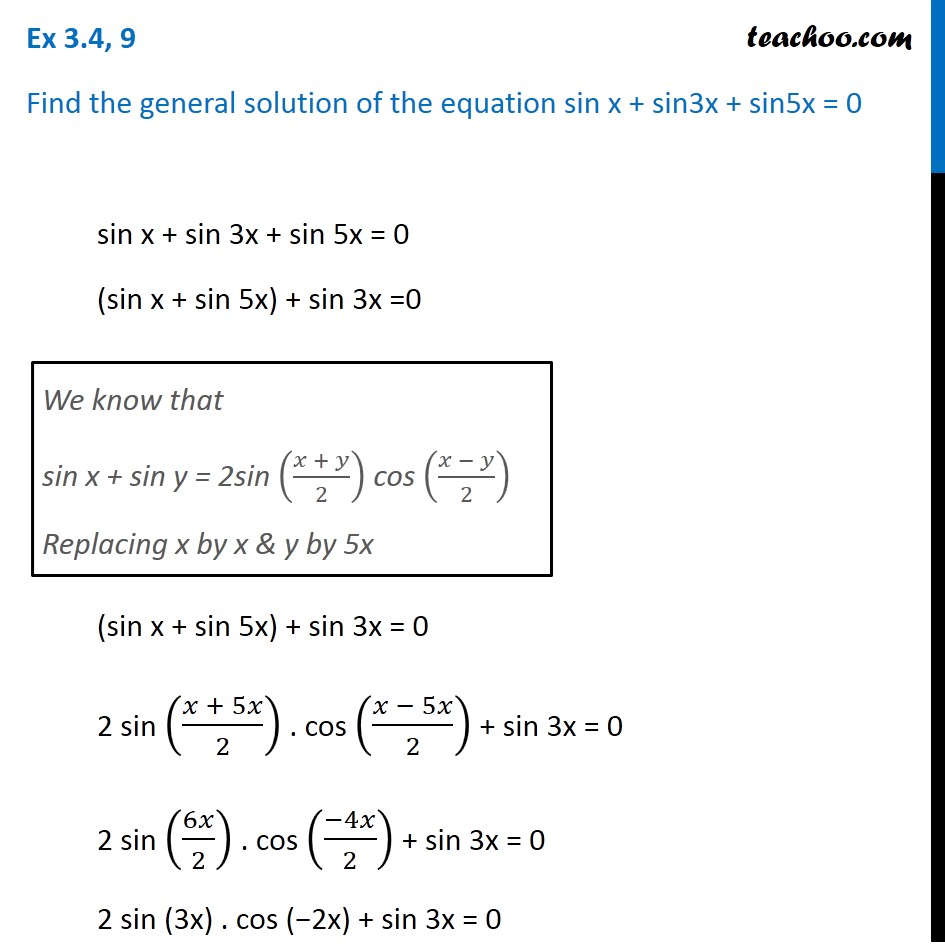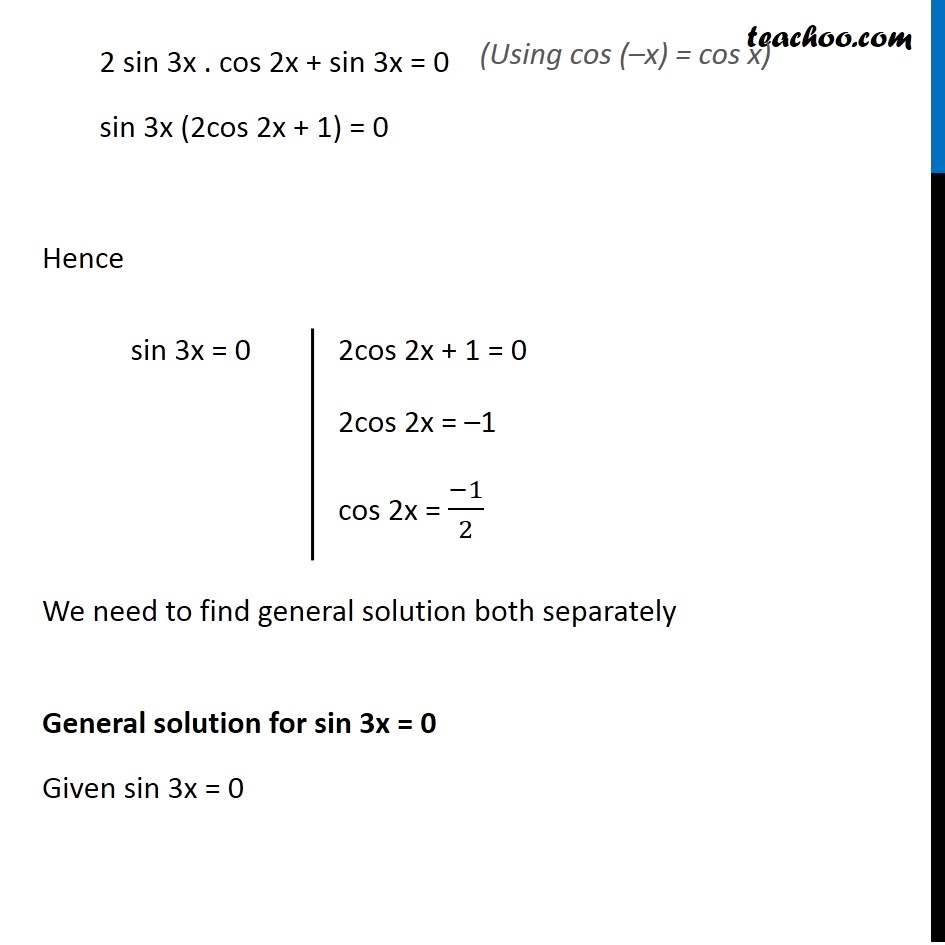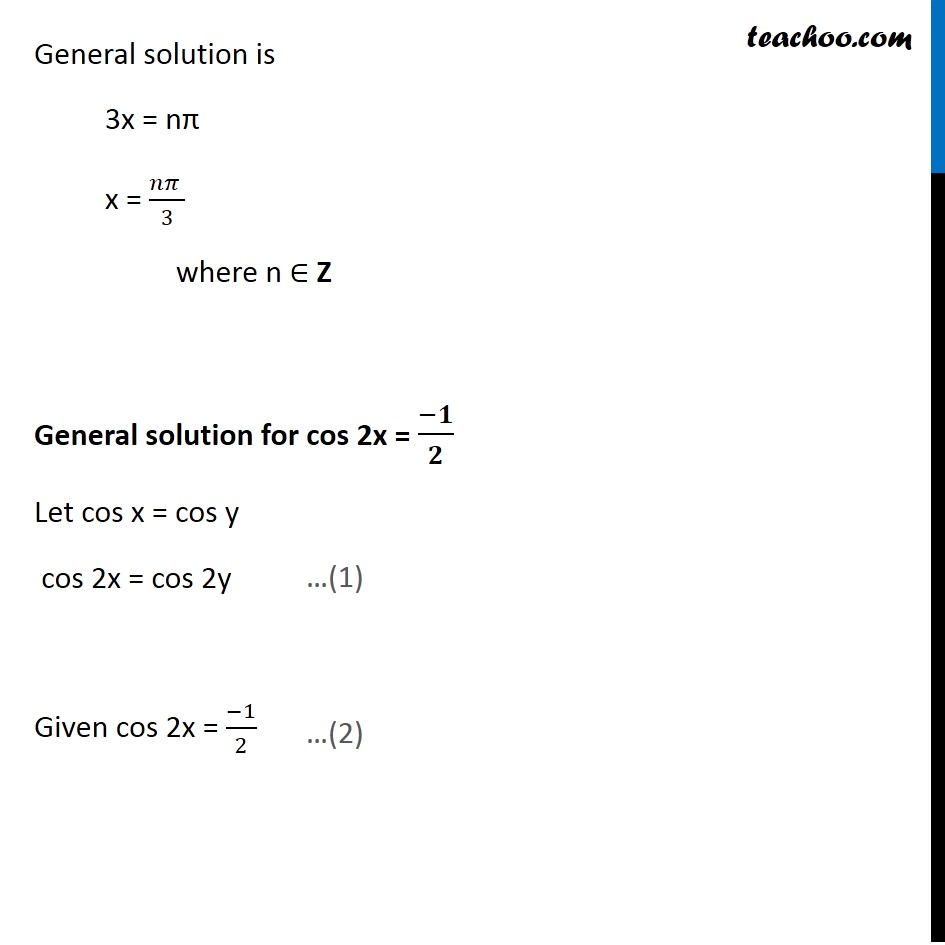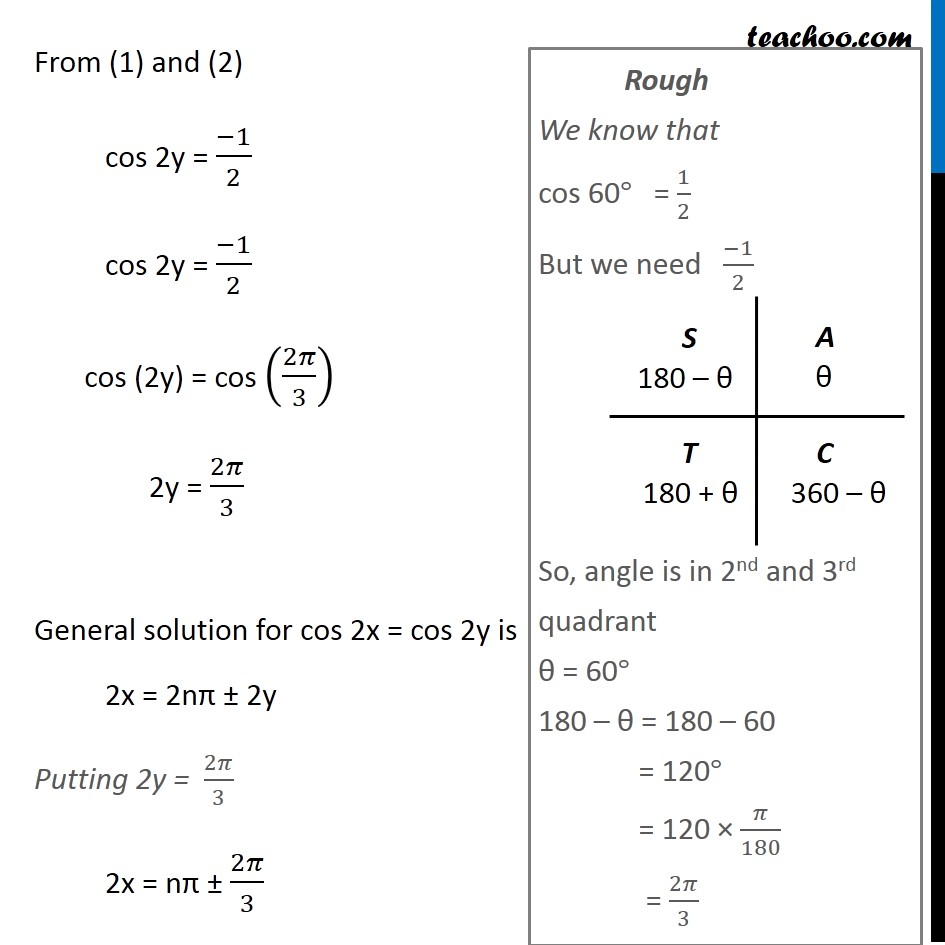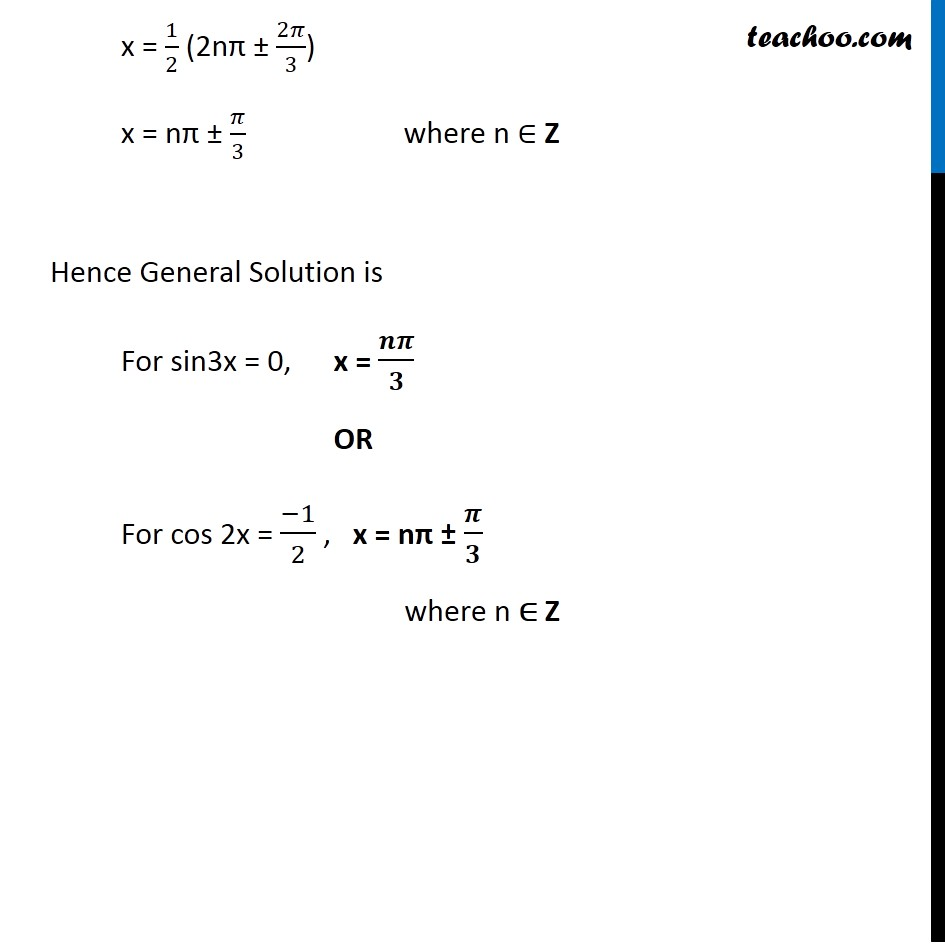Learn in your speed, with individual attention - Teachoo Maths 1-on-1 Class

### Transcript

Question 9 Find the general solution of the equation sin x + sin3x + sin5x = 0 sin x + sin 3x + sin 5x = 0 (sin x + sin 5x) + sin 3x =0 (sin x + sin 5x) + sin 3x = 0 2 sin ((𝑥 + 5𝑥)/2) . cos ((𝑥 − 5𝑥)/2) + sin 3x = 0 2 sin (6𝑥/2) . cos ((−4𝑥)/2) + sin 3x = 0 2 sin (3x) . cos (−2x) + sin 3x = 0 We know that sin x + sin y = 2sin ((𝑥 + 𝑦)/2) cos ((𝑥 − 𝑦)/2) Replacing x by x & y by 5x 2 sin 3x . cos 2x + sin 3x = 0 sin 3x (2cos 2x + 1) = 0 Hence We need to find general solution both separately General solution for sin 3x = 0 Given sin 3x = 0 sin 3x = 0 2cos 2x + 1 = 0 2cos 2x = –1 cos 2x = (−1)/2 General solution is 3x = nπ x = (𝑛𝜋 )/3 where n ∈ Z General solution for cos 2x = (−𝟏)/𝟐 Let cos x = cos y cos 2x = cos 2y Given cos 2x = (−1)/2 From (1) and (2) cos 2y = (−1)/2 cos 2y = (−1)/2 cos (2y) = cos (2𝜋/3) 2y = 2𝜋/3 General solution for cos 2x = cos 2y is 2x = 2nπ ± 2y Putting 2y = 2𝜋/3 2x = nπ ± 2𝜋/3 Rough We know that cos 60° = 1/2 But we need (−1)/2 So, angle is in 2nd and 3rd quadrant θ = 60° 180 – θ = 180 – 60 = 120° = 120 × 𝜋/180 = 2𝜋/3 x = 1/2 (2nπ ± 2𝜋/3) x = nπ ± 𝜋/3 where n ∈ Z Hence General Solution is For sin3x = 0, x = 𝒏𝝅/𝟑 OR For cos 2x = (−1)/2 , x = nπ ± 𝝅/𝟑 where n ∈ Z